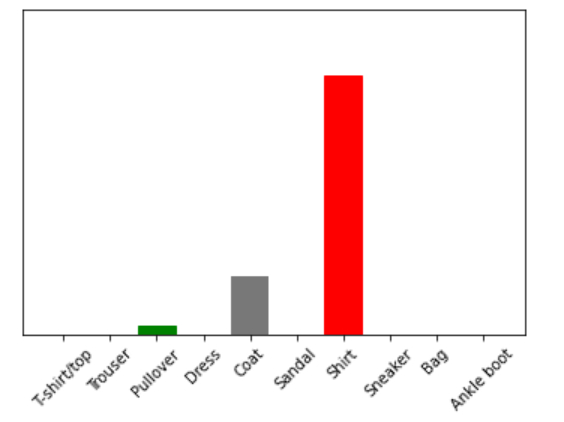# How can Tensorflow be used with Fashion MNIST dataset so that the trained model is used to predict a different image in Python?

PythonServer Side ProgrammingProgramming

Tensorflow is a machine learning framework that is provided by Google. It is an open-source framework used in conjunction with Python to implement algorithms, deep learning applications and much more. It is used in research and for production purposes. It has optimization techniques that help in performing complicated mathematical operations quickly. This is because it uses NumPy and multi-dimensional arrays. These multi-dimensional arrays are also known as ‘tensors’. The framework supports working with deep neural network.

The ‘tensorflow’ package can be installed on Windows using the below line of code −

pip install tensorflow

Tensor is a data structure used in TensorFlow. It helps connect edges in a flow diagram. This flow diagram is known as the ‘Data flow graph’. Tensors are nothing but multidimensional array or a list. They can be identified using three main attributes −

Rank − It tells about the dimensionality of the tensor. It can be understood as the order of the tensor or the number of dimensions in the tensor that has been defined.

Type − It tells about the data type associated with the elements of the Tensor. It can be a one dimensional, two dimensional or n dimensional tensor.

Shape − It is the number of rows and columns together.

The ‘Fashion MNIST’ dataset contains images of clothing of different kinds. It contains grayscale images of more than 70 thousand clothes that belong to 10 different categories. These images are of low resolution (28 x 28 pixels).

We are using the Google Colaboratory to run the below code. Google Colab or Colaboratory helps run Python code over the browser and requires zero configuration and free access to GPUs (Graphical Processing Units). Colaboratory has been built on top of Jupyter Notebook.

Following is the code snippet −

## EXample

print("An image from the test data is taken")
img = test_images
print("The dimensions of the image are ")
print(img.shape)
print("The image is added to batch where it is the only entity")
img = (np.expand_dims(img,0))
print("The dimensions of the image now ")
print(img.shape)

my_pred = probability_model.predict(img)
print(my_pred)

plot_value_array(1, my_pred, test_labels)
_ = plt.xticks(range(10), class_names, rotation=45)
np.argmax(my_pred)

## Output

An image from the test data is taken
The dimensions of the image are
(28, 28)
The image is added to batch where it is the only entity
The dimensions of the image now
(1, 28, 28)
[[8.0459216e-07 1.7074371e-09 2.6175227e-02 1.8855806e-07 1.7909618e-01
2.1126857e-06 7.9472500e-01 7.5104166e-11 4.7921480e-07 1.6657851e-10]]
6## Explanation

• The dimensions of the test image is displayed on the console

• The ‘expand_dims’ is optimized to work on a batch or collection of examples simultaneously.

• A single image is also added as a part of a list.

• The predict function returns a list of lists, where every list corresponds to an image in the batch data.

• The predictions for the image that we want is extracted and displayed on the console.

• It is visualized using ‘matplotlib’ as a bar graph.